Q&A - Ask Doubts and Get Answers

Sort by :
Q
represents change enthalpy  And  represents the change in internal energy. According to First law of thermodynamics, H and E are related as,
Engineering
139 Views   |

Let A and B be two sets containing 2 elements and 4 elements respectively. The number of subsets of AB having 3 or more elements is :

• Option 1)

211

• Option 2)

256

• Option 3)

220

• Option 4)

219

n(A) = 4, n(B) = 2

Number of sbsets having atlest 3 elements

Option 1)

211

Incorrect

Option 2)

256

Incorrect

Option 3)

220

Incorrect

Option 4)

219

Correct

View More
Engineering
440 Views   |

The length of a focal chord of the parabola y2 = 4ax at a distance b from the vertex is C. Then

• Option 1)

2a2 = bc

• Option 2)

a3 = b2c

• Option 3)

ac = b2

• Option 4)

b2c = 4a3

Find intersections A(x1, y1), B(x2, y2) of P & L Eliminate y from (1) & (2): m2x2 - 2am x + m2 a2 = 4ax  m2 x2-2a(m + 2) x + m2 a2 = 0                     ..............(3) x1, x2 are the roots, x1 + x2 = 2a (m+2)/m2 ;; x1 x2 = a2         .........(4) Eliminate x from (1) & (2): y = m(y2/4a) - ma my2 - 4a y-4a2 m = 0                        ..........(5) y1, y2 are the roots, y1 + y2 = 4a/m   ...
Engineering
136 Views   |

The inverse of the function     is

• Option 1)

• Option 2)

• Option 3)

• Option 4)

None of these

As we learnt in  Co - Domain of function - All possible outcomes for the function f(x) is known as co - domain unless not specified in question. -     Range of function - All possible values of  domain   is known as Range  -     Option 1) Option is correct Option 2) Option is incorrect Option 3) Option is incorrect Option 4) None of these Option is incorrect
Engineering
179 Views   |

Four identical electrical lamps are labelled 1.5V, 0.5A which describes the condition necessary for them to operate at normal brightness. A 12V battery of negligible internal resistance is connected to lamps as shown, then• Option 1)

The value of R for normal brightness of each lamp is

• Option 2)

The value of R for normal brightness of each lamp is

• Option 3)

Total power dissipated in circuit when all lamps are normally bright is 24W

• Option 4)

Power dissipated in R is 21W when all lamps are normally bright

V = IR    For normal brightness current through each bulb is 0.5 A Therefore, Total current = 2A Option 1) The value of R for normal brightness of each lamp is   Incorrect Option 2) The value of R for normal brightness of each lamp is Correct Option 3) Total power dissipated in circuit when all lamps are normally bright is 24W Incorrect Option 4) Power dissipated in R is 21W when all lamps...
Engineering
617 Views   |

When the rms voltages VL, VC and VR are  measured respectively across the inductor L, the capacitor C and the resistor R in a series  LCR  circuit  connected  to  an AC source, it is found that the ratio VL : VC : VR = 1 : 2 : 3. If the rms voltage of the AC source is 100 V, then VR is close to :

• Option 1)

50 V

• Option 2)

70 V

• Option 3)

90 V

• Option 4)

100 V

or   Option 1) 50 V Incorrect Option 2) 70 V Incorrect Option 3) 90 V Correct Option 4) 100 V Incorrect
Engineering
118 Views   |

The radius of the circle passing through the points (1,2) (5,2)and (5,-2) is

• Option 1)

• Option 2)

• Option 3)

• Option 4)

As we learnt in Equation of a circle - - wherein Circle with centre and radius .    On substituting the  value of  (x, y) as (1, 2), we get              ....................(1)  On substituting the  value of  (x, y) as (5, 2), we get         .........................(2) On substituting the  value of  (x, y) as (5, -2), we get        .......................(3) On subtracting  Eq. (1) - (2), we...
Engineering
104 Views   |

The radius of the circle passing through the points (1,2),(5,2) and (5,-2) is

• Option 1)

• Option 2)

• Option 3)

• Option 4)

As we learnt in

Equation of a circle -

- wherein

Circle with centre and radius .

On substituting the  value of  (x, y) as (1, 2), we get

....................(1)

On substituting the  value of  (x, y) as (5, 2), we get

.........................(2)

On substituting the  value of  (x, y) as (5, -2), we get

.......................(3)

On subtracting  Eq. (1) - (2), we get

h = 3

On subtacting Eq. (2) - Eq. (3), we get

k = 0

On

Option 1)

Incorrect

Option 2)

Incorrect

Option 3)

Incorrect

Option 4)

Correct

View More
Engineering
181 Views   |

Which one of the following complexes shows optical isomerism ?

• Option 1)

[Co(NH3)3Cl3]

• Option 2)

cis[Co(en)2Cl2]Cl

• Option 3)

trans[Co(en)2Cl2]Cl

• Option 4)

[Co(NH3)4Cl2]Cl

(en=ethylenediamine)

As discussed in   These molecules are optically active having absence of plane of symmetry.  This molecule has absence of plane of symmetry and hence option 2 is correct. Option 1)  [Co(NH3)3Cl3]   This option is incorrect. Option 2)  cis[Co(en)2Cl2]Cl   This option is correct. Option 3) trans[Co(en)2Cl2]Cl   This option is incorrect. Option...
Engineering
329 Views   |

3-Methyl-pent-2-ene on reaction with HBr in presence of peroxide forms an addition product. The number of possible stereoisomers for the product is :

• Option 1)

Two

• Option 2)

Four

• Option 3)

Six

• Option 4)

Zero

The product obtained has 2 stereocent x and molecube is unsymmetrical No of stereoisomers = hence total stereoisomers = 4   Option 1) Two This is incorrect option Option 2) Four This is correct option Option 3) Six This is incorrect option Option 4) Zero This is incorrect option
Engineering
295 Views   |

An organic compound A upon reacting with NH3 gives B .On heating ,B gives C .C in presence of KOH reacts  With Br2 to give CH3CH2NH2. A is :

• Option 1)• Option 2)• Option 3)• Option 4)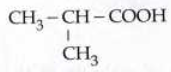As we learnt in  Hofmann Bromamide Degradation - Amines ( only primary ) can also be prepared by Hofmann degradation. In this method the amine will have one carbon atom less than the amide.  - wherein   Option 1) This option is correct Option 2) This option is incorrect Option 3) This option is incorrect Option 4) This option is incorrect
Engineering
476 Views   |

The final product formed when Methyl  amine is treated with NaNO2 and HCl is

• Option 1)

Diazomethane

• Option 2)

Methylalcohol

• Option 3)

Methylcyanide

• Option 4)

Nitromethane

As we learnt in  Reaction with Nitrous Acid Primary Amines - Primary amines react with nitrous acid to produce diazonium ion. - wherein     The values of ionization potential of an element decrease as the atomic size increases. Also, removal of electron from half or full filled atoms require more energy than expected with maximum in noble gases. Finally, IE increases along a period and...
Engineering
359 Views   |

When the concentration of alkyl halide is tripled and concentration of OH- is reduced to half, the rate of SN2 reaction increased by

• Option 1)

3 times

• Option 2)

1.5 times

• Option 3)

2 times

• Option 4)

6 times

As learnt in SN2 Substitution Nucleophilic bimolecular - These reactions proceed in only one step. - wherein     By increasing concentration of R-X 3 times and (OH-) reduced to half, the rate of SN2 reaction increased by 1.5 times   Option 1) 3 times Incorrect option Option 2) 1.5 times correct option Option 3) 2 times Incorrect option Option 4) 6 times Incorrect option
Engineering
297 Views   |

The formula of Prussian Blue is

• Option 1)

• Option 2)

• Option 3)

• Option 4)

None of these

As learnt in Test for Sulphur - The sodium fusion extract is acidified with acetic acid and bad acetate is added to sodium fusion. - wherein A block ppt of pbs confirm the sulphur.    the formula of Prussian blue is   Ferric ferrocyanide   Option 1) Incorrect option Option 2) correct option Option 3) Incorrect option Option 4) None of these Incorrect option
Engineering
351 Views   |

O - Chlorotoluene can undergo

(a) electrophillic aromatic substitution

(b) nucleophilic aromatic substitution

(c) nucleophilic aliphatic substitution

(d) free radical substitution

• Option 1)

Only (a)

• Option 2)

(a) and (d) only

• Option 3)

(a), (b) and (d)

• Option 4)

All of these

As we learnt in Halogenation reaction of haloarene - 1,2 - di chlorobenzene 1,4 - di chlorobenzene is obtained (major) - wherein ortho chlorotoluene can undergo electrophilic aromatic substitution at benzene ring, and Nucleophile aromatic and free radical substitute.   Option 1) Only (a) Incorrect option Option 2) (a) and (d) only Incorrect option Option 3) (a), (b) and (d) correct...
Engineering
157 Views   |

The structure of the major product formed in the given reaction is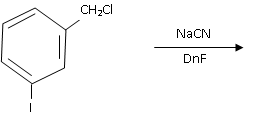• Option 1)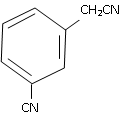• Option 2)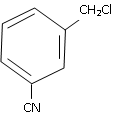• Option 3)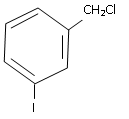• Option 4)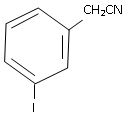As we learnt in SN2 Substitution Nucleophilic bimolecular - These reactions proceed in only one step. - wherein       Option 1) Incorrect Option 2) Incorrect Option 3) Incorrect Option 4) Correct
Engineering
410 Views   |

Which of the following compounds can exist both as a pair of enantiomers and a trans pair of enantiomers?

• Option 1)

• Option 2)

• Option 3)

• Option 4)

As we learnt in Optically active compound - Those compounds having capacity to rotate plane polarised light is called optically active compound. - wherein     This molecule has one chiral centre, hence, exist both as a pair of enantiomer and a trans pair of enantiomer.   Option 1) Incorrect Option 2) Correct Option 3) Incorrect Option 4) Incorrect
Engineering
143 Views   |

Which reagent will you use for the following reaction?

CH3 CH2 CH2 CH3    CH3 CH2 CH2 CH2 Cl   +   CH3 CH2 CH Cl CH3

• Option 1)

Cl2 / UV light

• Option 2)

NaCl + H2SO4

• Option 3)

Cl2 gas in dark

• Option 4)

Cl2 gas in the presence of iron in dark

As we learnt in Preparation of haloalkane by free radical halogenation of Alkanes - Alkanes undergo free radical substitution reaction when treated Cl2/Br2 in presence of sunlight. - wherein       Option 1) Cl2 / UV light Correct Option 2) NaCl + H2SO4 Incorrect Option 3) Cl2 gas in dark Incorrect Option 4) Cl2 gas in the presence of iron in dark Incorrect
Engineering
842 Views   |

Ethylidene Chloride on treatment with aq. KOH gives

• Option 1)

Acetaldehyde

• Option 2)

Ethylene Glycol

• Option 3)

Acetone

• Option 4)

Ethanol

As we learnt in Reaction of alkyl halide with aquous KOH - Alkyl halide when treated with Ag KOH Alcohol is obtained. -       Option 1) Acetaldehyde Correct Option 2) Ethylene Glycol Incorrect Option 3) Acetone Incorrect Option 4) Ethanol Incorrect
Engineering
354 Views   |

Alkyl flourides are synthesised by heating an alkyl chloride / bromide in presence of which of the following?

(a) CaF2

(b) COF2

(c) Hg2F2

(d) NaF

• Option 1)

(a) and (c)

• Option 2)

(b) and (c)

• Option 3)

(a) and (d)

• Option 4)

(d) and (b)

As discussed in Swarts reaction - This method is used to prepare alkyl fluorides from alkyl chloride or alkyl bromide, in the presence of AgF, Hg2F2, COF2. - wherein   Alkyl Fluorides are syntesised by heating alkyl chloride alkyl bromide in presence of ,   this  is called Swarts preparation Option 1) (a) and (c) This solution is incorrect Option 2) (b) and (c) This solution is...
Exams
Articles
Questions